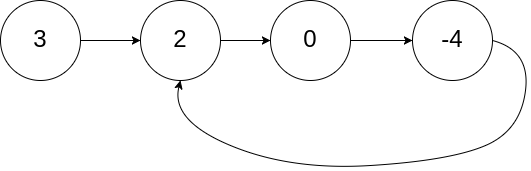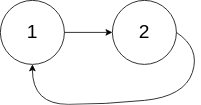## Algorithm

Problem Name: 141. Linked List Cycle

Given `head`, the head of a linked list, determine if the linked list has a cycle in it.

There is a cycle in a linked list if there is some node in the list that can be reached again by continuously following the `next` pointer. Internally, `pos` is used to denote the index of the node that tail's `next` pointer is connected to. Note that `pos` is not passed as a parameter.

Return `true` if there is a cycle in the linked list. Otherwise, return `false`.

Example 1:```Input: head = [3,2,0,-4], pos = 1
Output: true
Explanation: There is a cycle in the linked list, where the tail connects to the 1st node (0-indexed).
```

Example 2:```Input: head = [1,2], pos = 0
Output: true
Explanation: There is a cycle in the linked list, where the tail connects to the 0th node.
```

Example 3:```Input: head = , pos = -1
Output: false
Explanation: There is no cycle in the linked list.
```

Constraints:

• The number of the nodes in the list is in the range `[0, 104]`.
• `-105 <= Node.val <= 105`
• `pos` is `-1` or a valid index in the linked-list.

## Code Examples

### #1 Code Example with C Programming

```Code - C Programming```

``````
struct ListNode *n, *nn;

do {
n = n->next;           // one step
nn = nn->next;
if (nn) nn = nn->next;  // two steps
} while (nn && n != nn);

if (nn) return true;

return false;
}
``````
Copy The Code &

Input

cmd
head = [3,2,0,-4], pos = 1

Output

cmd
true

### #2 Code Example with C++ Programming

```Code - C++ Programming```

``````
class Solution {
public:
while(two && two->next){
one = one->next;
two = two->next->next;
if(one == two) return true;
}
return false;
}
};
``````
Copy The Code &

Input

cmd
head = [3,2,0,-4], pos = 1

Output

cmd
true

### #3 Code Example with Java Programming

```Code - Java Programming```

``````
public class Solution {
while (fast != null && fast.next != null) {
slow = slow.next;
fast = fast.next.next;
if (slow == fast) {
return true;
}
}
return false;
}
}
``````
Copy The Code &

Input

cmd
head = [1,2], pos = 0

Output

cmd
true

### #4 Code Example with Javascript Programming

```Code - Javascript Programming```

``````
const seen = []
return true
} else {
}
}
return false
};
``````
Copy The Code &

Input

cmd
head = [1,2], pos = 0

Output

cmd
true

### #5 Code Example with Python Programming

```Code - Python Programming```

``````
class Solution:
def hasCycle(self, head: ListNode) -> bool:
while slow != None and fast != None:
if slow == fast:
return True
slow, fast = slow.next, fast.next.next if fast.next else None
return False
``````
Copy The Code &

Input

cmd
head = , pos = -1false

Output

cmd
false

### #6 Code Example with C# Programming

```Code - C# Programming```

``````
namespace LeetCode
{
{
{

while (slow != fast)
{
if (fast == null || fast.next == null) return false;
slow = slow.next;
fast = fast.next.next;
}

return true;
}
}
}
``````
Copy The Code &

Input

cmd
head = , pos = -1

Output

cmd
false